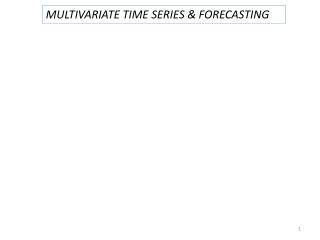DownloadDownload PresentationMULTIVARIATE TIME SERIES & FORECASTING

# MULTIVARIATE TIME SERIES & FORECASTING

Télécharger la présentation## MULTIVARIATE TIME SERIES & FORECASTING

- - - - - - - - - - - - - - - - - - - - - - - - - - - E N D - - - - - - - - - - - - - - - - - - - - - - - - - - -
##### Presentation Transcript

1. MULTIVARIATE TIME SERIES & FORECASTING

2. Vector ARMA models Stationarity if the roots of the equation are all greater than 1 in absolute value Then : infinite MA representation

3. Invertibility if the roots of the equation are all greater than 1 in absolute value ARMA(1,1)

4. If case of non stationarity: apply differencing of appropriate degree

5. VAR models (vector autoregressive models) are used for multivariate time series. The structure is that each variable is a linear function of past lags of itself and past lags of the other variables.

6. Vector AR (VAR) models Vector AR(p) model For a stationary vector AR process: infinite MA representation

7. The Yule-Walker equations can be obtained from the first p equations The autocorrelation matrix of Var(p) : decaying behavior following a mixture of exponential decay & damped sinusoid

8. Autocovariance matrix V:diagonal matrix The eigenvalues of determine the behavior of the autocorrelation matrix

9. Example 1. Data : the pressure reading s at two ends of an industrial furnace Expected: individual time series to be autocorrelated & cross-correlated Fit a multivariate time series model to the data 2.Identify model - Sample ACF plots - Cross correlation of the time series

10. Exponential decay pattern: autoregressive model & VAR(1) or VAR(2) Or ARIMA model to individual time series & take into consideration the cross correlation of the residuals

11. VAR(1) provided a good fit

12. ARCH/GARCH Models (autoregressive conditionally heteroscedastic) • -a model for the variance of a time series. • used to describe a changing, possibly volatile variance. • most often it is used in situations in which there may be short periods of increased variation. (Gradually increasing variance connected to a gradually increasing mean level might be better handled by transforming the variable.)

13. Not constant variance Consider AR(p)= model Errors: uncorrelated, zero mean noise with changing variance Model et2 as an AR(l) process white noise with zero mean & constant variance et: Autoregressive conditional heteroscedastic process of order l –ARCH(l)

14. Generalise ARCH model Consider the error: et: Generalised Autoregressive conditional heteroscedastic process of order k and l–GARCH(k,l)

15. S& P index • -Initial data: non stationary • Log transformation of the data • First differences of the log data Mean stable Changes in the variance ACF& PACF: No autocorrelation left in the data

16. ACF & PACF of the squared differences: ARCH (3) model